×

Search anything:

# Multilayer Perceptrons vs CNN

#### Machine Learning (ML) cnnGet this book -> Problems on Array: For Interviews and Competitive Programming

Multilayer Perceptron and CNN are two fundamental concepts in Machine Learning. When we apply activations to Multilayer perceptrons, we get Artificial Neural Network (ANN) which is one of the earliest ML models. CNN can later as an improvements to the limitations of ANN/ Multilayer perceptrons.

# Key Differences between ANN (Multilayer Perceptron) and CNN

• CNN is mostly used for Image Data, whereas it is better to use ANN on structural data
• CNN has less parameters and tries to reduce the dimensions of image whereas in case of ANN number of parameters depends on the data
• CNN is complex in nature whereas ANN is relatively simple compared to CNN
• CNN uses special Convolution and Pooling Layers whereas ANN is just a network of Neurons
• CNN is generally used for huge or bulky data as compared to ANN

# Introduction

Artificial Intelligence and Deep Learning are the hot trendings topics right now. Any company be it Google, financial institutions like Goldman Sachs or consulting firms like E&Y and Delloite, are trying their best to gain monopoly in Artificial Intelligence.Today we are going to discuss some important concepts in Deep Learning - Multilayer Perceptrons and Convolutional Neural Networks(CNN)
Before getting started let's discuss what a Perceptron and a Neuron is !

# Perceptron

A Perceptron is the simplest decision making algorithm. It has certain weights and takes certain inputs. The output of the Perceptron is the sum of the weights multiplied with the inputs with a bias added. Based on this output a Perceptron is activated. A simple model will be to activate the Perceptron if output is greater than zero. Thus, we can manipulate the weights and bias to get the desired ouput.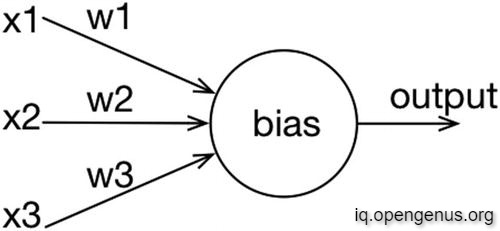A single Perceptron is very limited in scope, we therefore use a layer of Perceptrons starting with an Input Layer. For each subsequent layers, the output of the current layer acts as the input of the next layer. The last layer is called Output Layer and the layers in-between are called Hidden Layers. This is called a Multilayer Perceptron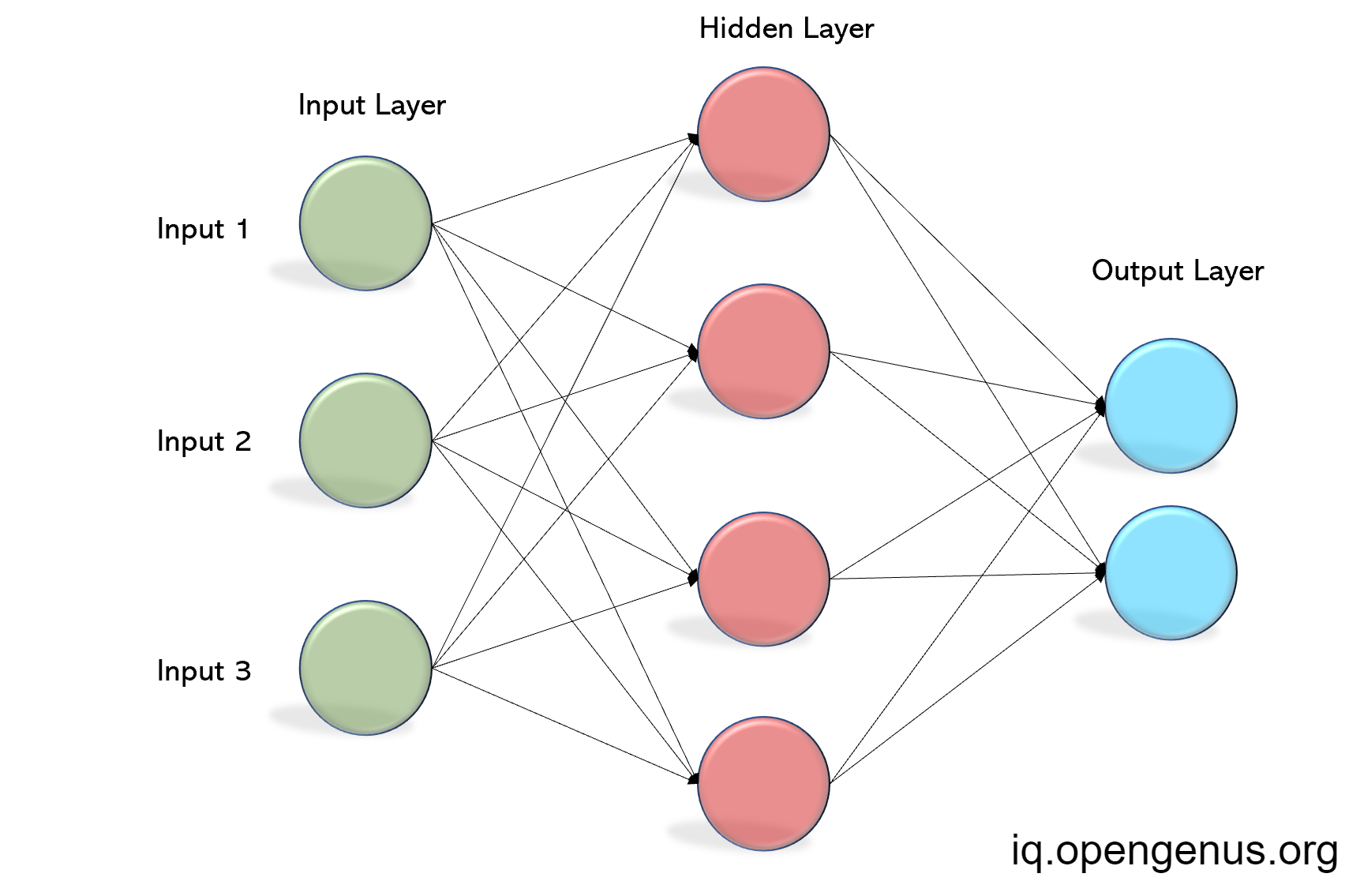When an activation function is applied to a Perceptron, it is called a Neuron and a network of Neurons is called Neural Network or Artificial Neural Network (ANN). Some examples of activation functions are Sigmoid Function and ReLU Function
A Neural Network looks the same as a Multilayered Perceptron. A neural network having more than 3 hidden layers is called a Deep Neural Network
In this article, Multilayer Perceptron and Neural Network will mean the same thing

# Problems with Neural Networks and need for CNN

In the beginning Neural Networks we used for all sorts of basic tasks like Regression and Classification. As the quantity of data increased the parameters of ANN also increased. With advancement in technology, Classification tasks were also required for image and text files but on using ANN, it was found that the computational powers sky-rocketed as the parameters increased to 100 thousands in numbers even for a small 8-bit image. Therefore, there was a need for an another type of Neural Network which would compensate for the sudden increase in computational powers required for applying Classifiction problems to Images and Text Files.

Let's see the problems ourselves !

Suppose we want to apply classifiction problems to a 16-bit image. A 2D-grayscale image would 16 * 16 = 256 pixels. Therefore, it will require a neural network with a input layer of 256 Neurons. Suppose, the image is colored then it will require 16 * 16 * 16 = 4096 Neurons in the input layer itself. Thus a huge amount of computational power is required for this task.

# Convolutional Neural Networks

CNN was fist introduced by Yann LeCun (current Vice President of Facebook AI) to classify handwritten digits based on their 20x20 pixel images.
CNN is mainly used to work with visual data and is mostly used in Robotics and Computer Vision.
We first are going to discuss the Convolution Layer.
An image is just a matrix of pixels. Instead of flattening the image, what CNN does is, it uses Kernels/Filter to read the patterns in the image. A Kernel is a small matrix where each cell has certain value with which the pixel value is multiplied and the convolved feature is extracted. Below is the visual representation of this !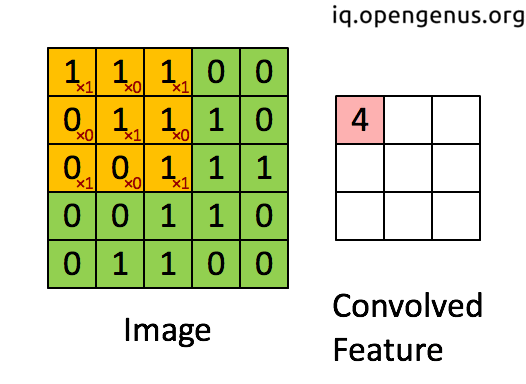To avoid loss of data a padding is applied on the corners (missing pixels are assumed 0) to prevent loss of data.
In additional to Convolutional Layer there is also a Pooling layer which further diminishes the dimensions of our image. A widely used Pooling layer is Max Pooling layer which takes the maximum value in current kernel as it slides the current layer.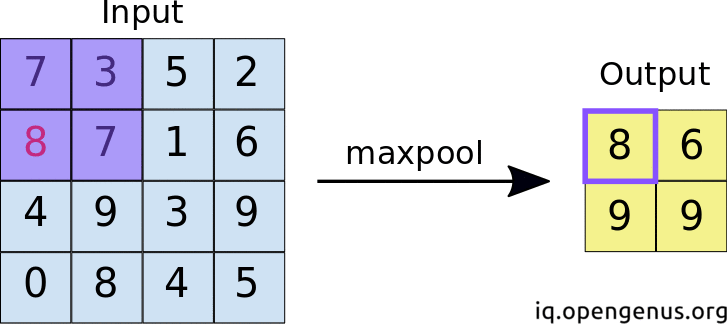After our image has reduced to a certain dimensions we are free to flatten our image and use an ANN as usual. Thus CNN save computation by using Convolution and Pooling Layers
Below is CNN to classify handwritten digits, similar to one proposed by Yann LeCunn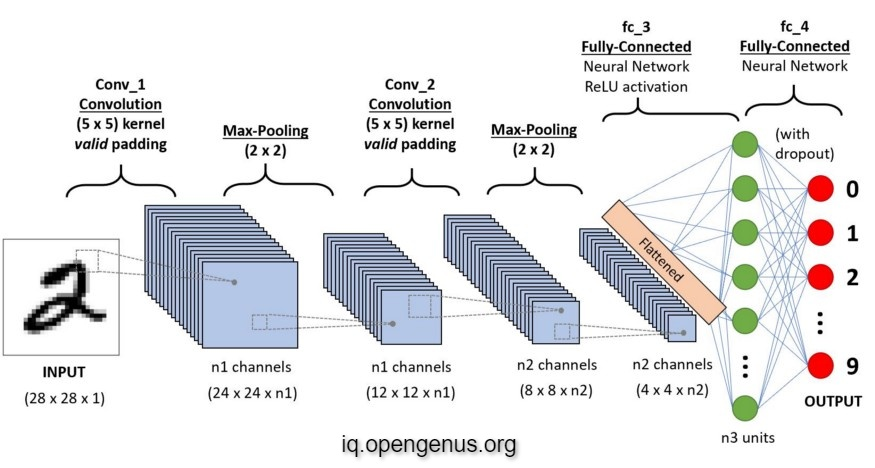CNN is a huge topic in itself and I have only given a brief description. This will do for now

# Key Differences

• CNN is mostly used for Image Data, whereas it is better to use ANN on structural data
• CNN has less parameters and tries to reduce the dimensions of image whereas in case of ANN number of parameters depends on the data
• CNN is complex in nature whereas ANN is relatively simple compared to CNN
• CNN uses special Convolution and Pooling Layers whereas ANN is just a network of Neurons
• CNN is generally used for huge or bulky data as compared to ANN

# Conclusion

In this article we saw some important Neural Networks the ANN and CNN. ANN are the traditional Neural Networks suitable for working with structured data. CNNs are relatively new are used for Image as well as Text data. CNN are most important research topic currently and is mainly used in Robotics and Computer Vision.